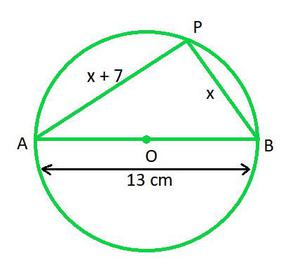GFG App
Open AppBrowser
Continue

# Class 10 RD Sharma Solutions – Chapter 8 Quadratic Equations – Exercise 8.10

### Question 1. The hypotenuse of a right triangle is 25 cm. The difference between the lengths of the other two sides of the triangle is 5 cm. Find the lengths of these sides.

Solution:

Let the length of other two sides of the triangle be x and y.

Therefore, according to the question,

x – y = 5

or,

x = y + 5

Now the two sides are of length y and y + 5.

Applying Pythagoras theorem on the right-angled triangle, we get,

252 = y2 + (y + 5)2

625 = y2 + y2 + 25 + 10y

2y2 + 25 + 10y = 625

2y2 + 10y = 625 – 25

2y2 + 10y – 600 = 0

y2 + 5y – 300 = 0

y2 + 20y – 15y – 300 = 0 [By middle term splitting]

y(y + 20) – 15(y + 20) = 0

(y – 15)(y + 20) = 0

y -15 = 0 or y + 20 = 0

y = 15 or y = -20

As the length of a side is positive, we will take y = 15.

Therefore,

x = y + 5

x = 15 + 5

x = 20

The two sides of the triangle are 15 cm and 20 cm.

### Question 2. The diagonal of a rectangular field is 60 meters more than the shorter side. If the longer side is 30 meters more than the shorter side, find the sides of the field.

Solution:

Let the shorter side of the rectangle be x.

Now, according to the question, length of rectangle is x + 60 and length of the longer side of rectangle is x + 30.

Now, we will apply Pythagoras theorem on the right-angled triangle formed by the diagonal and the two sides,

(x + 60)2 = (x + 30)2 + x2

x2 + 3600 + 120x = x2 + 900 + 60x + x2

3600 – 900  = x2 + 60x – 120x

2700 = x2 – 60x

x2 – 60x – 2700 = 0

x2 – 90x + 30x – 2700 = 0

x(x – 90) + 30(x – 90) = 0

(x + 30)(x – 90) = 0

x + 30 = 0 or x – 90 = 0

x = -30 or x = 90

We will take positive side, i.e. x = 90

Now,

length of shorter side is 90 m

length of diagonal is 90 + 60 = 150 m

length of longer side is 90 + 30 = 120 m

### Question 3. The hypotenuse of a right triangle is 3√10 cm. If the smaller leg is tripled and the longer leg doubled, new hypotenuse will be 9√5 cm. How long are the legs of the triangle?

Solution:

Let the smaller and longer side of the triangle be x and y.

Now applying Pythagoras theorem, we get,

(3√10)2 = x2 + y2

x2 + y2 = 90     ……… (i)

Now the smaller and longer sides are tripled and doubled respectively,

Therefore, the new sides of the triangle will be 3x and 2y.

Applying Pythagoras theorem,

(9√5)2 = (3x)2 + (2y)2

9x2 + 4y2 = 405     ……… (ii)

Now multiplying (i) by 4 and then subtracting (i) from (ii), we get

9x2 – 5x2 + 4y2 – 4y2= 405 – 360

5x2 = 45

x2 = 9

x = 3

Substituting value of x in (i),

9 + y2 = 90

y2 = 81

y = 9

Length of smaller side is 3 cm.

Length of longer side is 9 cm.

### Question 4. A pole has to be erected at a point on the boundary of a circular park of diameter 13 metres in such a way that the difference of its distances from two diametrically opposite fixed gates A and B on the boundary is 7 metres. Is it possible to do so? If yes, at what distances from the two gates should the pole be erected?

Solution:In the circle, let P be the pole on the circumference of the circle and points A and B be the two diametrically opposite fixed gates.

Let length of PB be x.

Given,

PA – PB = 7

PA = x + PB

PA = x + 7

The triangle PQR is a right-angled triangle at P, as AB is the diameter of the circle.

Now applying Pythagoras theorem,

AB2 = PA2 + PB2

132 = (x + 7)2 + x2

169 = x2 + 49 + 14x + x2

169 = 2×2 + 14x + 49

2x2 + 14x + 49 – 169 = 0

2x2 + 14x – 120 = 0

x2 + 7x – 60 = 0

x2 + 12x – 5x – 60 = 0

x(x + 12) – 5(x + 12) = 0

(x – 5)(x + 12) = 0

x = 5 or x = -12

Only x = 5 is possible.

PB = 5 m

PA = 5 + 7 = 12 m

P should be erected at a distance of 5 m from PB and 12 m from PA.

My Personal Notes arrow_drop_up